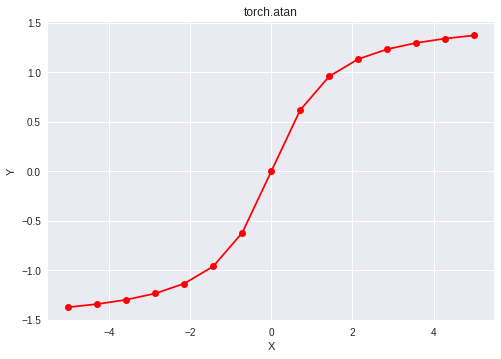GeeksforGeeks App
Open AppBrowser
Continue

PyTorch is an open-source machine learning library developed by Facebook. It is used for deep neural network and natural language processing purposes.

The function `torch.atan()` provides support for the inverse tangent function in PyTorch. It gives the output in radian form. The input type is tensor and if the input contains more than one element, element-wise inverse tangent is computed.

Syntax: torch.atan(x, out=None)

Parameters:
x: Input tensor
name (optional): Output tensor

Return type: A tensor with the same type as that of x.

Code #1:

## Python3

 `# Importing the PyTorch library``import` `torch`` ` `# A constant tensor of size 6``a ``=` `torch.FloatTensor([``1.0``, ``-``0.5``, ``3.4``, ``0.2``, ``0.0``, ``-``2``])``print``(a)`` ` `# Applying the inverse tan function and``# storing the result in 'b'``b ``=` `torch.atan(a)``print``(b)`

Output:

```tensor([ 1.0000, -0.5000,  3.4000,  0.2000,  0.0000, -2.0000])
tensor([ 0.7854, -0.4636,  1.2847,  0.1974,  0.0000, -1.1071])
```

Code #2: Visualization

## Python3

 `# Importing the PyTorch library``import` `torch`` ` `# Importing the NumPy library``import` `numpy as np`` ` `# Importing the matplotlib.pyplot function``import` `matplotlib.pyplot as plt`` ` `# A vector of size 15 with values from -5 to 5``a ``=` `np.linspace(``-``5``, ``5``, ``15``)`` ` `# Applying the inverse tangent function and``# storing the result in 'b'``b ``=` `torch.atan(torch.FloatTensor(a))`` ` `print``(b)`` ` `# Plotting``plt.plot(a, b.numpy(), color ``=` `'red'``, marker ``=` `"o"``) ``plt.title(``"torch.atan"``) ``plt.xlabel(``"X"``) ``plt.ylabel(``"Y"``) `` ` `plt.show()`

Output:

```tensor([-1.3734, -1.3416, -1.2978, -1.2341, -1.1342, -0.9601, -0.6202,  0.0000,
0.6202,  0.9601,  1.1342,  1.2341,  1.2978,  1.3416,  1.3734])
```My Personal Notes arrow_drop_up